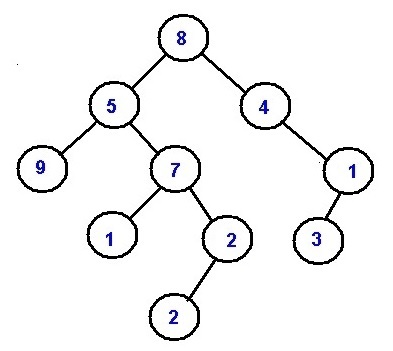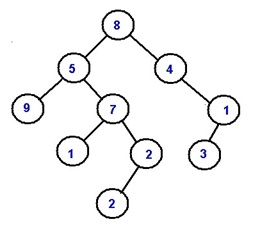Q:

# Sum of all numbers formed by root to leaf path

In this article, we are going to see how to calculate root to leaf path sums? This problem has been featured in Google interview.
Submitted by Radib Kar, on March 28, 2019

Problem statement:

Given a binary tree, where every node value is a number between 0-9. Find the sum of all the numbers which are formed from root to leaf paths.

Example:All possible roots to leaf paths in this tree are:

```    8->5->9 //number formed=859
8->5->7->1 //number formed=8571
8->5->7->2->2 //number formed=85722
8->4->1->3//number formed=8413

Sum: 859+8571+85722+8413=103565```

The idea is quite similar as we did for the problem all root to leaf paths (read: All root to leaf paths

Here instead of printing them we are forming a number from the digits on the path and storing the numbers for addition.

Algorithm:

Pre-requisite:

Input binary tree rootlist astack st

```    FUNCTION printpathrecursively(Node* current_node, list  a){
1.  base case
IF(current_node ==NULL)
return;
END IF
2.  pushcurrent_node ->data to the stack;
IF current_node is leaf node //leaf node reached
Form number from the digits stored in the stack st
Append the formed number to the list a
END IF
3.  printpathrecursively(current_node ->left,a);//recur for left subtree
printpathrecursively(current_node ->right,a);//recur for right subtree
END FUNCTION
```

Sum all the numbers stored in the list a and print it.

N.B: Remember here the list parameter must be passed by reference to store all the numbers formed from the digits on the root-to-leaf path

In the main function create an empty list a, and call printpathrecursively(root, a, st);

Example with explanation:```    In main we call printpathrecursively(root, a, st)
---------------------------------------------------
printpathrecursively(8, a, st)
current node not NULL
push 8 to stack st
stack: 8
current node is not leaf node
call to printpathrecursively(8->left, a, st)
call to printpathrecursively(8->right, a, st)
---------------------------------------------------

printpathrecursively(8->left, a, st):
=printpathrecursively(5, a, st)
current node not NULL
push 5 to stack st
stack: 8,5
current node is not leaf node
call to printpathrecursively(5->left, a, st)
call to printpathrecursively(5->right, a, st)
---------------------------------------------------

printpathrecursively(5->left, a, st):
=printpathrecursively(9, a, st)
current node not NULL
push 9 to stack st
stack: 8, 5, 9
current node is leaf node
form the number from the stack
generated number is 859
append 859 to list a
call to printpathrecursively(9->left, a, st)
call to printpathrecursively(9->right, a, st)
call to printpathrecursively(9->left, a, st)
---------------------------------------------------

=printpathrecursively(NULL, a, st)
current node NULL

At printpathrecursively(9, a, st)
call to printpathrecursively(9->right, a, st)
---------------------------------------------------

=printpathrecursively(NULL, a, st)
current node NULL
At printpathrecursively(9, a, st)
Control reaches end of the void function
Thus control returns to the callee
function  printpathrecursively(5, a, st)
---------------------------------------------------

printpathrecursively(5->right, a, st):
=printpathrecursively(7, a, st)
current node not NULL
push 7 to stack st
stack: 8, 5, 7
current node is not leaf node

call to printpathrecursively(7->left, a, st)
call to printpathrecursively(7->right, a, st)
This can be carried out until control gets back to main
which called function printpathrecursively(root, a, st)
```

You can do rest by your own to have much more clear idea about how the program is actually working.

C++ Implementation:

``````#include <bits/stdc++.h>
using namespace std;

class Node{ //tree node
public:
int data;
Node *left;
Node *right;
};

void pathsum(Node* root, stack<int> a,vector<long long int> &digits){
//stack st same as stack a in code
//vector<int> digits in code same as list a
if(!root)
return;
a.push(root->data);
if(!root->left && !root->right){
//code to form numbers from the stack
long long int num=0;
int p=1;
stack<int> a1=a;
while(!a1.empty()){
num+=p*a1.top();
a1.pop();
p*=10;

}
digits.push_back(num); //append number to list
}

pathsum(root->left,a,digits);
pathsum(root->right,a,digits);

}

long long treePathsSum(Node *root)
{
stack<int> a;
vector<long long int> digits;
pathsum(root,a,digits);

long long int sum=0;
for(int i=0;i<digits.size();i++)
sum+=digits[i];

return sum;
}
Node* newnode(int data){  //creating new nodes
Node* node = (Node*)malloc(sizeof(Node));
node->data = data;
node->left = NULL;
node->right = NULL;
return(node);
}

int main() {
//**same tree is builted as shown in example**
cout<<"tree in the example is build here"<<endl;
//building the tree like as in the example
Node *root=newnode(8);
root->left= newnode(5);
root->right= newnode(4);
root->right->right=newnode(1);
root->right->right->left=newnode(3);
root->left->left=newnode(9);
root->left->right=newnode(7);
root->left->right->left=newnode(1);
root->left->right->right=newnode(2);
root->left->right->right->left=newnode(2);

cout<<"all root to leaf path sum is......"<<endl;
treePathsSum(root);
return 0;
}
``````

Output

```tree in the example is build here
all root to leaf path sum is......
103565```